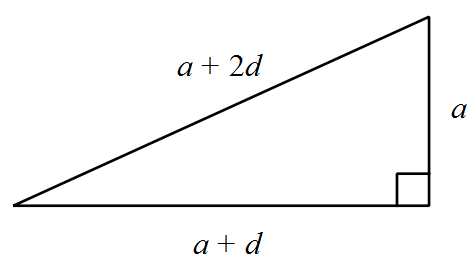# Are They In Progression?

Geometry Level 1The sides of a right triangle are $a$, $a+d$ and $a+2d$ with $a$ and $d$ both positive. What is the value of $a:d$?

Note: Image drawn not up to scale.

×